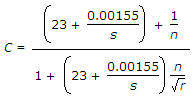# Civil Engineering - Waste Water Engineering - Discussion

### Discussion :: Waste Water Engineering - Section 5 (Q.No.4)

4.

The value of Chezy's constantis used in

 [A]. Chezy's formula [B]. Bazin's formula [C]. Kutter's [D]. Manning's formula.

Explanation:

No answer description available for this question.

 Shubh said: (Jun 9, 2020) The given equation is Kutter's equation. And it is used in the Chezy equation. So the answer should be A.